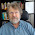## 03 June 2012

### A Tale of Two Dice

Let’s do a little experiment.Take two dice, one six-sided, the other ten-sided. If you don’t have the dice and there isn’t a game shop handy, you can always use Excel to simulate the experiment. We’re going to throw both dice many times and each time we’re going to record the maximum value of the two sides that turn up. After a few hundred throws, we’ll calculate the average of the values we’ve recorded.

But do we need to do the calculation? We know that the average of the six-sided throws is 3.5 and that the average of the ten-sided throws is 5.5. The maximum of 3.5 and 5.5 is 5.5.

But let's do the calculation anyway. What we get is an average of about 6.1. That can’t be! So we do it again. And again the average is about 6.1.

So what's going on here? The common sense explanation is that, since you always take the larger of the two values, the combined average should be bigger. How much bigger?

More generally, is there a general-purpose mathematical/statistical function that will produce the probability distribution that's the maximum of two probability distributions? The dice problem is easy – it involves the simplest distribution there is. And yet ...

I dredged the internet looking for a formula and came up empty – except for this valiant effort: http://www.cecs.uci.edu/~papers/iccad06/papers/3C_4.pdf

These guys find a formula that works for a very special shape of distribution that may or may not match the real world and, if I read it right, they force the result into the same shape.

The irony in this paper is that they use simulation to validate the formula. If the simulation results are the measure of the formula's validity, why not just simulate and be done with it, especially since simulation solves the problem for any shape of distribution?

That's one reason I say "Don't formulate. Simulate!"

#### 3 comments:

1.I've developed a method for calculating value of information in Monte Carlo simulation (non-decision tree) that uses the exact approach you describe here for almost the exact situation you describe: find the average of the pairwise sample max of two distributions.

2.Hi Rob,

It's what I do to model converging workflows in a project model - except I keep the distribution as input to what follows or, if it's the final result, an aid to decisions about project resourcing.

It may need more explanation at first but I find a CDF graph much more useful than an average.

3.I hardly ever report average values to clients. All results on objective functions are given as CDFs or percentile bands across time series.